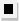Chapter 13.CR, Problem 54E### Algebra and Trigonometry (MindTap ...

4th Edition
James Stewart + 2 others
ISBN: 9781305071742

#### Solutions

Chapter
Section### Algebra and Trigonometry (MindTap ...

4th Edition
James Stewart + 2 others
ISBN: 9781305071742
Textbook Problem

# 4 9 - 5 4Sums of Arithmetic and Geometric Sequences Determine whether the expression is a partial sum of an arithmetic or geometric sequence. Then find the sum. ∑ k = 0 8 7 ( 5 ) k / 2

To determine

Whether the expression k=087(5)k/2 is partial sum of arithmetic or geometric sequence. Then find the sum.

Explanation

Given:

The expression is given as,

k=087(5)k/2

Approach:

Check if the terms in the expression have a common difference or a common ratio.

Calculation:

The summation can be expanded as:

7+7(5)1/2+7(5)1+7(5)3/2+7(5)2+7(5)5/2+7(5)3+7(5)7/2+7(5)4

Calculate the common difference.

7(5)1/27=8.657(5)17(5)1/2=19.35

So there is no common difference.

Calculate the common ratio.

7(5)1/2÷7=(5)1/27(5)1÷7(5)1/2=(5)1/27(5)3/2÷7(5)1=(5)1/2

This proves that the given expression is a partial sum of a geometric sequence with first term a=7, common ratio, r=(5)1/2

### Still sussing out bartleby?

Check out a sample textbook solution.

See a sample solution

#### The Solution to Your Study Problems

Bartleby provides explanations to thousands of textbook problems written by our experts, many with advanced degrees!

Get Started